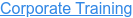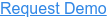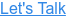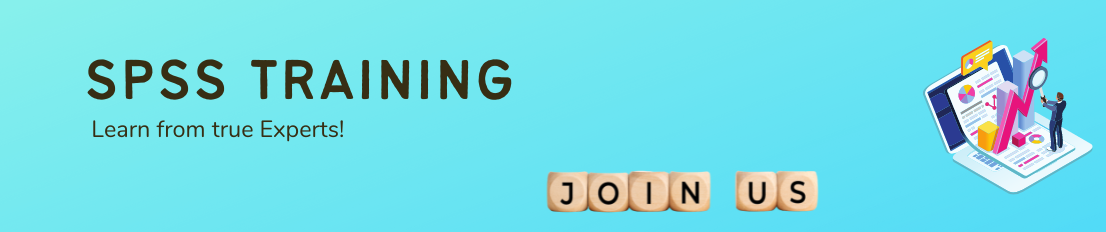#### 15+ Categories. 500+ IT Courses to Learn.by Sachin, on Jul 19, 2022 10:34:13 PM## Ans

SPSS is short for Statistical Package for the Social Sciences, and it's used by various kinds of researchers for complex statistical data analysis. The SPSS software package was created for the management and statistical analysis of social science data.

## Ans

SPSS (Statistical Package for the Social Sciences), also known as IBM SPSS Statistics, is a software package used for the analysis of statistical data. Although the name of SPSS reflects its original use in the field of social sciences, its use has since expanded into other data markets.

## Ans

There arthree types of SPSS files that we will use during this class: data files, which end in . sav; syntax files, which end in . sps; and output files, which end in . spv.

## Ans

Regression technique is used to assess the strength of a relationship between one dependent and independent variable(s). It helps in predicting value of a dependent variable from one or more independent variable.

## Ans

Creating tables and charts containing frequency counts or summary statistics over (groups of) cases and variables. running inferential statistics such as ANOVA, regression and factor analysis. saving data and output in a wide variety of file formats.## Ans

T-tests are used to compare the mean scores of two groups of people or conditions. There are two types of t-tests: Independent-samples t-test: compare the mean scores of two different groups of people or conditions. Paired-samples t-test: compare the mean scores for the same group of people on two different occasions.

## Ans

Each window is associated with specific tasks and types of SPSS files. The windows include the Data Editor, Output Viewer, Syntax Editor, Pivot Table Editor, Chart Editor, and Text Output Editor.

## Ans

The first variable (constant) represents the constant, also referred to in textbooks as the Y interceptthe height of the regression line when it crosses the Y axis. In other words, this is the predicted value of science when all other variables are 0.

## Ans

SPSS prints something called the R-square change, which is just the improvement in R-square when the second predictor is added. The R-square change is tested with an F-test, which is referred to as the F-change. A significant F-change means that the variables added in that step signficantly improved the prediction.

## Ans

Correlation is a statistical technique that shows how strongly two variables are related to each other or the degree of association between the two. For example, if we have the weight and height data of taller and shorter people, with the correlation between them, we can find out how these two variables are related.

## Ans

Quantitative Results. Statistical Analysis. Analysis of Variance, i.e. ANOVA in SPSS, is used for examining the differences in the mean values of the dependent variable associated with the effect of the controlled independent variables, after taking into account the influence of the uncontrolled independent variables.

## Ans

Adjusted R2 is a corrected goodness-of-fit (model accuracy) measure for linear models. It identifies the percentage of variance in the target field that is explained by the input or inputs. R2 tends to optimistically estimate the fit of the linear regression.

## Ans

The advantages of using SPSS as a software package compared to other are: SPSS is a comprehensive statistical software. Many complex statistical tests are available as a built in feature. Interpretation of results is relatively easy.

## Ans

2-tailed) – The p-value is the two-tailed probability computed using the t distribution. It is the probability of observing a t-value of equal or greater absolute value under the null hypothesis. For a one-tailed test, halve this probability.

## Ans

The parameter β (the regression coefficient) signifies the amount by which change in x must be multiplied to give the corresponding average change in y, or the amount y changes for a unit increase in x. In this way it represents the degree to which the line slopes upwards or downwards.

## Ans

In other fields, the standards for a good R-Squared reading can be much higher, such as 0.9 or above. In finance, an R-Squared above 0.7 would generally be seen as showing a high level of correlation, whereas a measure below 0.4 would show a low correlation.

## Ans

Pearson correlation: Pearson correlation evaluates the linear relationship between two continuous variables. Spearman correlation: Spearman correlation evaluates the monotonic relationship. The Spearman correlation coefficient is based on the ranked values for each variable rather than the raw data.

## Ans

Dependent variables are variables that change if there is a change in another variable of interest. For instance, I might hypothesise that children from low-earning families struggle at school, compared to children from more affluent backgrounds. School performance, in this hypothesis, is a dependent variable.

## Ans

To test the internal consistency, you can run the Cronbach's alpha test using the reliability command in SPSS, as follows: RELIABILITY /VARIABLES=q1 q2 q3 q4 q5. You can also use the drop-down menu in SPSS, as follows: From the top menu, click Analyze, then Scale, and then Reliability Analysis.

## Ans

A Dependent List: The continuous numeric variables you wish to analyze. B Factor List: (Optional) Categorical variables to subset the analysis by. The procedure will produce individual summaries of the numeric variable with respect to each category.

## Ans

SPSS provides data analysis for descriptive and bivariate statistics, numeral outcome predictions and predictions for identifying groups. The software also provides data transformation, graphing and direct marketing features. The software interface displays open data similarly to a spreadsheet in its main view.

## Ans

A null hypothesis is a precise statement about a population that we try to reject with sample data. We don't usually believe our null hypothesis (or H0) to be true. However, we need some exact statement as a starting point for statistical significance testing.

## Ans

• Pearson Correlation Coefficient.
• Linear Correlation Coefficient.
• Sample Correlation Coefficient.
• Population Correlation Coefficient.

## Ans

The normal distribution peaks in the middle and is symmetrical about the mean. Data does not need to be perfectly normally distributed for the tests to be reliable. Checking normality in SPSS. Data: The SPSS dataset 'NormS' contains the variables used in this sheet including the exercises.

## Ans

Each mean square is calculated by dividing the sum of square by its degrees of freedom. The fifth column gives the F ratio. It is calculated by dividing mean square between-groups by mean square within-groups.

## Ans

Negative Numbers. In a binary number, the bit furthest to the left is called the most significant bit (msb) and the bit furthest to the right is called the least significant bit (lsb).

## Ans

Calculate MSB = ( individual sample size n ) times ( sample variance of the means ) • Calculate degrees of freedom for numerator/MSB = df1 = (number of groups – 1).

## Ans

Skewness is a measure of symmetry, or more precisely, the lack of symmetry. A distribution, or data set, is symmetric if it looks the same to the left and right of the center point. Kurtosis is a measure of whether the data are heavy-tailed or light-tailed relative to a normal distribution.

## Ans

Step1: Turning the data to long format

Step2: Merge with the original data matching the variable friend in current data. Rename the variables and save the data by id.

Step3: Check whether focal and id are a pair of reciprocal friends.

Step4: Aggregate the long data to a single focal per row and merge back into the original data set.

## Ans

If you are using SPSS variation 14 or later, you can open it as a data record.

Select File - > Open - > Data… and after that for Files of Type select the reasonable sas data record make; by then select the report from the summary and snap Open. That is all to it.

With SPSS language structure, use ‘get sas’ to peruse in a SAS data record.

get sas data='C:datastates.sas7bdat'